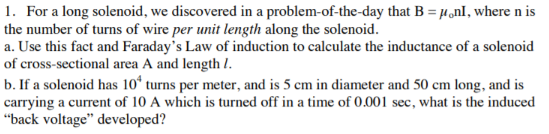# 1. For a long solenoid, we discovered in a problem-of-the-day that B = µ,nl, where n isthe number of turns of wire per unit length along the solenoid.a. Use this fact and Faraday's Law of induction to calculate the inductance of a solenoidof cross-sectional area A and length I.b. If a solenoid has 10ʻ turns per meter, and is 5 cm in diameter and 50 cm long, and iscarrying a current of 10 A which is turned off in a time of 0.001 sec, what is the induced"back voltage" developed?

Question
18 viewshelp_outlineImage Transcriptionclose1. For a long solenoid, we discovered in a problem-of-the-day that B = µ,nl, where n is the number of turns of wire per unit length along the solenoid. a. Use this fact and Faraday's Law of induction to calculate the inductance of a solenoid of cross-sectional area A and length I. b. If a solenoid has 10ʻ turns per meter, and is 5 cm in diameter and 50 cm long, and is carrying a current of 10 A which is turned off in a time of 0.001 sec, what is the induced "back voltage" developed? fullscreen
check_circle

Step 1

(a)

Consider l be the length of the solenoid.

The expression for n in term of N, total number of terms are:

Step 2

From faraday law of induction, the formula for the flux through a solenoid is:

Step 3

Also, as mentioned in the question, the ex...

### Want to see the full answer?

See Solution

#### Want to see this answer and more?

Solutions are written by subject experts who are available 24/7. Questions are typically answered within 1 hour.*

See Solution
*Response times may vary by subject and question.
Tagged in

### Magnetic Effects of Current